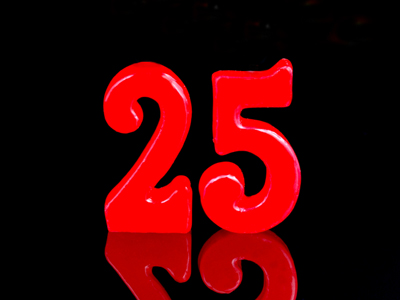If you multiply 5 by itself, you get 25.

# Properties of Numbers 1

This Math quiz is called 'Properties of Numbers 1' and it has been written by teachers to help you if you are studying the subject at middle school. Playing educational quizzes is a fabulous way to learn if you are in the 6th, 7th or 8th grade - aged 11 to 14.

It costs only \$12.50 per month to play this quiz and over 3,500 others that help you with your school work. You can subscribe on the page at Join Us

The properties of numbers are things like factors or multiples. Learning the properties of numbers is all about understanding the relationships between them. For example, do you know the multiples and factors of numbers? Let's take the number 8. Its multiples are all of the numbers in the 8 times table from 0 upwards. Its factors are the numbers that can be multiplied together to make 8, which are 1, 2, 4 and 8. How much have you learned about the properties of numbers?

1.
Which of these numbers is a multiple of 4?
5,698
2,463
4,441
2,548
We can easily work this out as 2,548 is the only number where the last 2 digits are divisible by 4
2.
What is a factor?
A factor is a whole number that divides into another whole number
A factor is a whole number that can be divided by 6
A factor is a number that cannot be divided
A factor is the product when a number is multiplied by itself
For example, 3 and 2 are both factors of 6
3.
What is 5 squared?
5
10
15
25
5 x 5 = 25
4.
What is a square number?
A number that can be divided by 4
A number that is a multiple of 6 and 7
The product when a number is multiplied by itself
A number that is written in a square
For example 3 x 3 = 9 therefore 9 is the square number of 3
5.
Which of these numbers is a multiple of 7?
15
22
35
37
35 is 5 x 7
6.
Which number has the factors of 1, 2, 4, 8 and 16?
8
16
24
36
Every number is a factor of itself as it is itself multiplied by 1
7.
How many pairs of factors will give 12?
1
3
6
12
They are 1 x 12, 2 x 6 and 3 x 4
8.
Which number multiplied by itself gives 49?
3
6
5
7
9.
How do we know that a number is a multiple of 4?
It ends in 2, 4 or 8
The last two digits are divisible by 4
It is also a multiple of 8
There is no way of knowing
We can ignore numbers in the 100s column as 100 is itself divisible by 4
10.
What is the square number of 6?
6
12
36
60
36 is 6 x 6
Author:  Amanda Swift